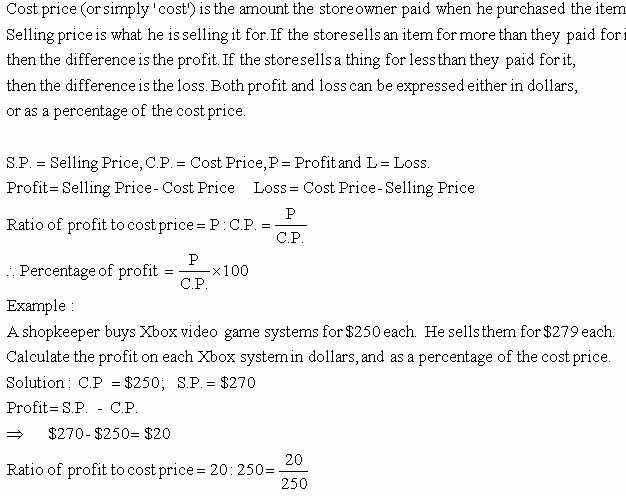kwizNET Subscribers, please login to turn off the Ads!
 Email us to get an instant 20% discount on highly effective K-12 Math & English kwizNET Programs!

#### Online Quiz (WorksheetABCD)

Questions Per Quiz = 2 4 6 8 10

### Grade 6 - Mathematics8.6 Finding Profit PercentagePercentage of profit = (20/250) x 100 = 0.08 = 8% Directions: Answer the following questions. Also write at least ten examples of your own.
 Q 1: Cost price = \$6500 and selling price = \$9000, what is the profit percentage?38 6/13%6 13/38%13 6/38% Q 2: Cost price = \$5000 and selling price = \$6000, what is the profit percentage?20%15%30% Q 3: Cost price = \$2500 and selling price = \$3000, what is the profit percentage?25%15%20% Q 4: Cost price = \$5500 and selling price = \$7000, what is the profit percentage?11 3/27%3 11/27%27 3/11% Q 5: Cost price = \$3000 and selling price = \$3300, what is the profit percentage?30%15%10% Q 6: Cost price = \$4000 and selling price = \$4500, what is the profit percentage?2 1/12%1 2/12%12 1/2% Q 7: Cost price = \$3500 and selling price = \$3700, what is the profit percentage?5 5/7%6 5/7%7 5/7% Q 8: Cost price = \$3600 and selling price = \$4000, what is the profit percentage?11 1/9%1 9/11%9 1/11% Question 9: This question is available to subscribers only! Question 10: This question is available to subscribers only!

#### Subscription to kwizNET Learning System offers the following benefits:

• Unrestricted access to grade appropriate lessons, quizzes, & printable worksheets
• Instant scoring of online quizzes
• Progress tracking and award certificates to keep your student motivated
• Unlimited practice with auto-generated 'WIZ MATH' quizzes
• Child-friendly website with no advertisements
• Choice of Math, English, Science, & Social Studies Curriculums
• Excellent value for K-12 and ACT, SAT, & TOEFL Test Preparation
• Get discount offers by sending an email to discounts@kwiznet.com

 Quiz Timer